4.9.3.4 Starting at a Found Node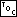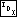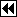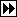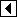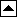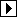Sometimes it is convenient to begin a traversal at a particular item in a tree. This function works in the same was as bst_find(), but records parent pointers in the traverser structure as it descends the tree.

```67. <BST traverser search initializer 67> =
void *bst_t_find (struct bst_traverser *trav, struct bst_table *tree, void *item) {
struct bst_node *p, *q;

assert (trav != NULL && tree != NULL && item != NULL);
trav->bst_table = tree;
trav->bst_height = 0;
trav->bst_generation = tree->bst_generation;
for (p = tree->bst_root; p != NULL; p = q)     {
int cmp = tree->bst_compare (item, p->bst_data, tree->bst_param);

if (cmp < 0)         q = p->bst_link;
else if (cmp > 0)         q = p->bst_link;
else /* cmp == 0 */         {
trav->bst_node = p;
return p->bst_data;
}

if (trav->bst_height >= BST_MAX_HEIGHT)         {
bst_balance (trav->bst_table);
return bst_t_find (trav, tree, item);
}
trav->bst_stack[trav->bst_height++] = p;
}

trav->bst_height = 0;
trav->bst_node = NULL;
return NULL;
}
```

This code is included in 63.